Find free online Chemistry Topics covering a broad range of concepts from research institutes around the world.

## The Integrated Rate Equation

We have just learnt that the rate of change of concentration of the reactant is directly proportional to that of concentration of the reactant. For a general reaction, A → product. The rate law is Rate = $$\frac{-d[A]}{dt}$$ = k[A]x

Where k is the rate constant, and x is the order of the reaction. The above equation is a differential equation, $$\frac{-d[A]}{dt}$$, so it gives rate at any instant. However, using the above expression, we cannot answer questions such as how long will it take for a specific concentration of A to be used up in the reaction? What will be the concentration of reactant after a time ‘t’?. To answer such questions, we need the integrated form of the above rate law which contains time as a variable.

Integrated Rate Law for a First Order Reaction

A reaction whose rate depends on the reactant concentration raised to the first power is called a first order reaction. Let us consider the following first order reaction,

A → Product

Rate law can be expressed as
Rate = k[A]1
Where, k is the fist order rate constant.
$$\frac{-d[A]}{dt}$$ = k[A]1
⇒ $$\frac{-d[A]}{[A]}$$ = k dt …………… (1)

Integrate the above equation between the limits of time t = 0 and time equal to t, while the concentration varies from the initial concentration [A0] to [A] at the later time.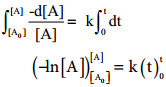– ln[A] – (- In[A0]) = k (t-0)
– ln[A] + In[A0] = kt
ln($$\frac{\left[\mathrm{A}_{0}\right]}{[\mathrm{A}]}$$) = kt ……….. (2)

This equation is in natural logarithm. To convert it into usual logarithm with base 10, we have to multiply the term by 2.303. 2.303 log ($$\frac{\left[\mathrm{A}_{0}\right]}{[\mathrm{A}]}$$) = kt
k = $$\frac{2.303}{t}$$log($$\frac{\left[\mathrm{A}_{0}\right]}{[\mathrm{A}]}$$) ………… (3)
Equation (2) can be written in the form y = mx + c as below
ln[A0]-ln[A] = kt
ln[A] = ln[A0]-kt
⇒ y = c + mx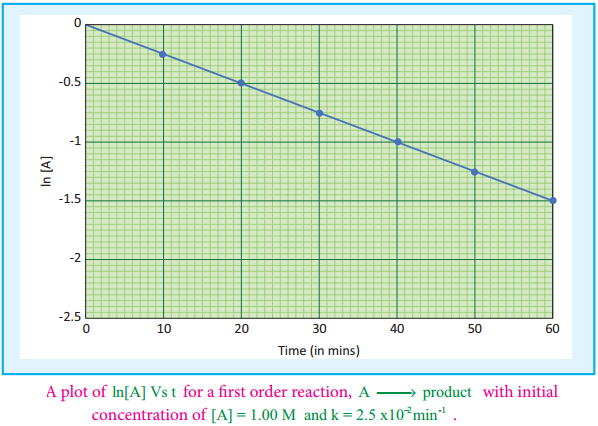If we follow the reaction by measuring the concentration of the reactants at regular time interval ‘t’, a plot of ln[A] against ‘t’ yields a straight line with a negative slope. From this, the rate constant is calculated. Examples for the first order reaction

(i) Decomposition of Dinitrogen Pentoxide

N2O5(g) → 2NO2(g) + $$\frac{1}{2}$$O2(g)

(ii) Decomposition of Sulphurylchloride

SO2Cl2(l) → SO2(g) + Cl2(g)

(iii) Decomposition of the H2O2 in aqueous solution; H2O2 → H2O(l) + $$\frac{1}{2}$$O2(g)

(iv) Isomerisation of Cyclopropane to Propene.

Pseudo First Order Reaction:

Kinetic study of a higher order reaction is difficult to follow, for example, in a study of a second order reaction involving two different reactants; the simultaneous measurement of change in the concentration of both the reactants is very difficult.

To overcome such difficulties, A second order reaction can be altered to a first order reaction by taking one of the reactant in large excess, such reaction is called pseudo first order reaction. Let us consider the acid hydrolysis of an ester,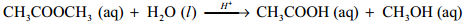Rate = k [CH3COOCH3] [H2O]

If the reaction is carried out with the large excess of water, there is no significant change in the concentration of water during hydrolysis. i.e.,concentration of water remains almost a constant.

Now, we can define k [H2O] = k’; Therefore the above rate equation becomes
Rate = k'[CH3COOCH3]
Thus it follows first order kinetics.

Integrated Rate law for a Zero Order Reaction:

A reaction in which the rate is independent of the concentration of the reactant over a wide range of concentrations is called as zero order reactions. Such reactions are rare. Let us consider the following hypothetical zero order reaction.

A → product
The rate law can be written as,
Rate = k[A]°
$$\frac{-d[A]}{dt}$$ = k(1) (∴[A]° = 1)
⇒ -d[A] = k dt

Integrate the above equation between the limits of [A°] at zero time and [A] at some later time ‘t’,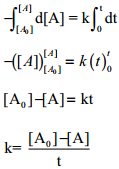Equation (2) is in the form of a straight line y = mx + c
i.e., [A] = – kt + [A°]
⇒ y = c + mx

A plot of [A] Vs time gives a straight line with a slope of – k and y – intercept of [A°].

Fig 7.4: A plot of [A] Vs time for a zero order reaction A → product with initial concentration of [A] = 0.5M and k = 1.5 × 10-2mol-1L-1min-1Examples for a Zero Order Reaction:

1. Photochemical reaction between H2 and I2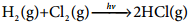2. Decomposition of N2O on hot Platinum Surface

N2O(g) ⇄ N2(g) + $$\frac{1}{2}$$O2(g)

3. Iodination of Acetone in Acid Medium is Zero Order With Respect to Iodine.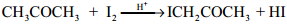Rate = k [CH3COCH3][H+]